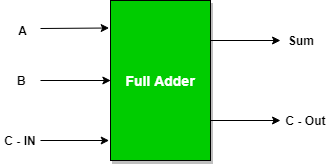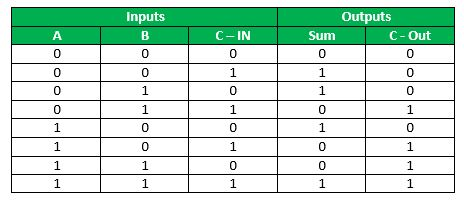# Python program to implement Full Adder

• Difficulty Level : Basic
• Last Updated : 09 Jun, 2021

Prerequisite : Full Adder in Digital Logic
Given three inputs of Full Adder A, B,C-IN. The task is to implement the Full Adder circuit and Print output i.e sum and C-Out of three inputs.

Full Adder : A Full Adder is a logical circuit that performs an addition operation on three one-bit binary numbers. The full adder produces a sum of the three inputs and carry value.

Attention reader! Don’t stop learning now. Get hold of all the important CS Theory concepts for SDE interviews with the CS Theory Course at a student-friendly price and become industry ready.Logical Expression :

```SUM  =  C-IN  XOR  ( A XOR B )
C-0UT= A B + B C-IN + A C-IN```

Truth Table :Examples :

```Input : 0 1 1
Output: Sum=0, C-Out=1```

According to logical expression Sum= C-IN XOR (A XOR B )  i.e 1 XOR (0 XOR 1) =0 , C-Out= A B + B C-IN + A C-IN  i.e.,  0 AND 1 + 1 AND 1 + 0 AND 1 = 1

```Input : 1 0 0
Output: Sum=1, C-Out=0```

Approach :

• We take three inputs A ,B and C-in .
• Applying C-IN XOR (A XOR B ) gives the value of sum
• Applying  A B + B C-IN + A C-IN gives the value of C-Out

Below is the implementation :

## Python3

 `# python program to implement full adder` `# Function to print sum and C-Out``def` `getResult(A, B, C):` `    ``# Calculating value of sum``    ``Sum` `=` `C ^ (A ^ B)``    ``C``    ``# Calculating value of C-Out``    ``C_Out ``=` `Bin``&(``not``(A ^ B))| ``not``(A)&B` `    ``# printing the values``    ``print``(``"Sum = "``, ``Sum``)``    ``print``(``"C-Out = "``, C_Out)`  `# Driver code``A ``=` `0``B ``=` `0``C ``=` `1``# passing three inputs of fulladder as arguments to get result function``getResult(A, B, C)`

Output :

```Sum =  1
C-Out =  0```
My Personal Notes arrow_drop_up